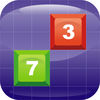# Matris - mathematical game

Alex Baranovich
Matris is a mathematical game similar to Tetris. The 6х9 playing field is being filled with cubes with digits from 1 to …
\$0.99
##### Details
Matris is a mathematical game similar to Tetris. The 6х9 playing field is being filled with cubes with digits from 1 to 9. Your task is to liberate the field from the cubes that disappear if the sum of the adjacent cubes is equal to or multiple of 10. The game has 7 modes: Line - the cubes disappear if the sum of any quantity of the cubes is divisible by 10 horizontally. 2 figures - the cubes disappear if the sum of 2 cubes is equal to 10 horizontally or vertically.. 3 figures - the cubes disappear if the sum of 3 cubes is multiple of 10 vertically or horizontally. 4 figures - the cubes disappear if the sum of 4 cubes is multiple of 10 vertically or horizontally. Square 2x2 - the cubes disappear if their amount in any 2x2 square is multiple of 10. Square 3x3 - the cubes disappear if their amount in any 3x3 square is multiple of 10. Rectangle 2х3 - the cubes disappear if their amount in any 2x3 rectangle is multiple of 10.
Information
Seller:Alex Baranovich
Genre:Board, Puzzle
Release:Nov 19, 2014
Updated:Dec 18, 2018
Version:2.0
Size:32.1 MB
TouchArcade Rating:Unrated
User Rating:Unrated
Your Rating:unrated
Compatibility:HD Universal